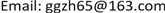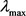﻿ 基于校院两级督导制度的教学质量评价指标体系构建研究 Research on the Construction of Teaching Quality Evaluation Index System Based on the Two-Level Supervision System of the University and College

Creative Education Studies
Vol. 07  No. 03 ( 2019 ), Article ID: 30974 , 8 pages
10.12677/CES.2019.73062

Research on the Construction of Teaching Quality Evaluation Index System Based on the Two-Level Supervision System of the University and College

Guizhen Gao

Dean’s Office, Henan University of Technology, Zhengzhou HenanReceived: June 4th, 2019; accepted: June 19th, 2019; published: June 26th, 2019ABSTRACT

Teaching supervision and teaching evaluation are important links in the teaching quality assurance system of colleges and universities. At present, although many colleges and universities have established the two-level supervision system, there is still no perfect evaluation index system and no consistent evaluation standard in evaluating teachers’ teaching work. In this study, the evaluation index system of the university and college was constructed, the weight was determined by expert investigation, and the index system was tested by fuzzy comprehensive evaluation method. The study found that the weight of the university-level supervisor evaluation was 0.354, and the weight of the college-level supervisor evaluation was 0.646. The teaching quality evaluation system supervised by the university and the college is scientific and effective, reasonable suggestions for implementing the index system are given. This study can promote the teaching supervision work to be carried out more scientifically and reasonably, and effectively improve the teaching level of teachers in colleges and universities.

Keywords:University and College, Teaching Supervision, Evaluation Index1. 引言

2. 校院两级教学督导的组织形式

3. 教学指标体系构建

3.1. 建立指标体系

3.2. 确定权重

1) 建立层次结构模型

2) 构造判断矩阵

$\begin{array}{l}{E}_{1}=\left(\begin{array}{cc}1& 1/2\\ 2& 1\end{array}\right),{E}_{2}=\left(\begin{array}{cc}1& 1\\ 1& 1\end{array}\right),{E}_{3}=\left(\begin{array}{cc}1& 1/3\\ 3& 1\end{array}\right),{E}_{4}=\left(\begin{array}{cc}1& 1/3\\ 3& 1\end{array}\right),\\ {E}_{5}=\left(\begin{array}{cc}1& 1/2\\ 2& 1\end{array}\right),{E}_{6}=\left(\begin{array}{cc}1& 1\\ 1& 1\end{array}\right),{E}_{7}=\left(\begin{array}{cc}1& 1/2\\ 2& 1\end{array}\right),{E}_{8}=\left(\begin{array}{cc}1& 1/2\\ 2& 1\end{array}\right)\end{array}$= 2，特征向量为(0.4472, 0.8944)T，对特征向量进行归一化处理，可得(0.333, 0.667)T，接下来结算一致性指标CI，由公式 $CI=\left({\lambda }_{\mathrm{max}}-n\right)/\left(n-1\right)$ 可得，

$CI=\left(2-2\right)/\left(2-1\right)=0$

CR = 0 < 0.10，因此指标层的判断矩阵符合一致性要求，因此，第一个专家的B1权重为0.333，B2权重为0.667。Table 4. B1 Factor item discriminant matrix, weight and CR valueTable 5. B2 Factor item discriminant matrix, weight and CR value

3.3. 模糊综合评价

1) 建立高等院校教师教学质量评估综合因素评价集合V

$\begin{array}{c}V=\left\{{B}_{1},{B}_{2}\right\}\\ =\left\{校级教学督导评价，院级教学督导评价\right\}\end{array}$

2) 质量标准化各因素的权重分配，根据上一小节对权重的确定，

3) 校级和院级教学督导教学质量评价等级

4) 建立评价矩阵Ri

${R}_{1}=\left(\begin{array}{ccccc}0.8& 0.1& 0.1& 0& 0\\ 0.9& 0.1& 0& 0& 0\\ 0.8& 0.2& 0& 0& 0\end{array}\right)$

${R}_{2}=\left(\begin{array}{ccccc}0.9& 0.1& 0& 0& 0\\ 0.9& 0.1& 0& 0& 0\\ 0.8& 0.2& 0& 0& 0\\ 0.8& 0.2& 0& 0& 0\\ 0.9& 0.1& 0& 0& 0\end{array}\right)$

5) 求各因素评价矩阵

${B}_{1}={C}_{1}×{R}_{1}=\left(\begin{array}{ccc}0.443& 0.170& 0.387\end{array}\right)×{R}_{1}=\left(\begin{array}{ccccc}0.8& 0.1& 0.1& 0& 0\\ 0.9& 0.1& 0& 0& 0\\ 0.8& 0.2& 0& 0& 0\end{array}\right)$

6) 建立总评价矩阵B

$B=\left(\begin{array}{ccccc}0.817& 0.139& 0.044& 0& 0\\ 0.871& 0.129& 0& 0& 0\end{array}\right)$

7) 求风险评价矩阵C

$\begin{array}{c}C=A×B=\left(\begin{array}{cc}0.354& 0.646\end{array}\right)\left(\begin{array}{ccccc}0.817& 0.139& 0.044& 0& 0\\ 0.871& 0.129& 0& 0& 0\end{array}\right)\\ =\left(\begin{array}{ccccc}0.852& 0.133& 0.016& 0& 0\end{array}\right)\end{array}$

8) 对工程造价风险评价矩阵C进行归一化处理

$C=\left(\begin{array}{ccccc}0.851& 0.133& 0.016& 0& 0\end{array}\right)$

9) 求系统总得分

$\begin{array}{c}f={C}_{1}×{S}_{1}+{C}_{2}×{S}_{2}+{C}_{3}×{S}_{3}+{C}_{4}×{S}_{4}+{C}_{5}×{S}_{5}\\ =0.851×90+0.133×80+0.016×70+0×60+0×50\\ =88.35\end{array}$

4. 建议

5. 结论

2017年度河南省高等教育教学改革研究与实践一般项目(2017SJGLX300)。

Research on the Construction of Teaching Quality Evaluation Index System Based on the Two-Level Supervision System of the University and College[J]. 创新教育研究, 2019, 07(03): 351-358. https://doi.org/10.12677/CES.2019.73062

1. 1. 周志国. 地方本科院校校院两级管理机制研究[J]. 教育与职业, 2014(3): 27-29.

2. 2. 刘天佐, 周清明. 高等学校构建校院两级管理模式若干问题[J]. 教育研究, 2005(11): 39-43.

3. 3. 刘亚荣, 李志明, 唐宁, 韩东平, 韩景义, 肖刚. 高校校院两级管理模式研究[J]. 教育与经济, 2010(2): 12-15.

4. 4. 刘亚荣, 高建广, 梅强, 张金刚, 李华, 计建炳, 孙毅. 我国高校实行校院两级管理体制改革的调研报告[J]. 国家教育行政学院学报, 2008(3): 68-75.

5. 5. 都光珍. 高校校院两级管理体制改革的对策思考[J]. 国家教育行政学院学报, 2011(12): 16-20.

6. 6. 李古月, 李亚伟. 高校校院两级教学督导体系研究与实践[J]. 山东商业职业技术学院学报, 2016, 16(5): 19-21+45.

7. 7. 李萍莉. 全面构建两级管理体制下的教学督导与评价体系[J]. 扬州大学学报(高教研究版), 2006, 10(5): 83-85.

8. 8. 方绮联. 高等学校校院两级教学督导体系的探讨[J]. 重庆邮电大学学报(社会科学版), 2012, 24(5): 157-160.

9. 9. 李和生, 倪海儿, 王鸿飞. 校院两级管理制下院教学督导的实践与认识[J]. 宁波大学学报(教育科学版), 2006, 28(1): 52-55.